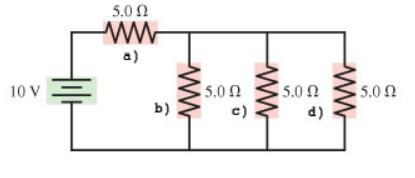# Problem: 1. Find the current through resistor a) in the figure. (Figure 1) Express your answer to two significant figures and include the appropriate units.2. Find the potential difference across resistor a) in the figure.3. Find the current through resistor b) in the figure.4. Find the potential difference across resistor b) in the figure.5. Find the current through resistor c) in the figure.6. Find the potential difference across resistor c) in the figure.7. Find the current through resistor d) in the figure.8. Find the potential difference across resistor d) in the figure 2.

###### FREE Expert Solution

Equivalent resistance for resistors in parallel:

$\overline{)\frac{\mathbf{1}}{{\mathbf{R}}_{\mathbf{eq}}}{\mathbf{=}}\frac{\mathbf{1}}{{\mathbf{R}}_{\mathbf{1}}}{\mathbf{+}}\frac{\mathbf{1}}{{\mathbf{R}}_{\mathbf{2}}}{\mathbf{+}}{\mathbf{.}}{\mathbf{.}}{\mathbf{.}}{\mathbf{+}}\frac{\mathbf{1}}{{\mathbf{R}}_{\mathbf{n}}}}$

Equivalent resistance for resistors in series:

$\overline{){{\mathbf{R}}}_{{\mathbf{eq}}}{\mathbf{=}}{{\mathbf{R}}}_{{\mathbf{1}}}{\mathbf{+}}{{\mathbf{R}}}_{{\mathbf{2}}}{\mathbf{+}}{\mathbf{.}}{\mathbf{.}}{\mathbf{.}}{\mathbf{+}}{{\mathbf{R}}}_{{\mathbf{n}}}}$

The potential difference across a resistor:

$\overline{){\mathbf{V}}{\mathbf{=}}{\mathbit{i}}{\mathbf{R}}}$

1.

Resistors b, c, and d are in parallel.

Therefore, 1/Rbcd = 1/5.0 + 1/5.0 + 1/5.0 = 3/5

Rbcd = 1.67Ω

88% (89 ratings)###### Problem Details1. Find the current through resistor a) in the figure. (Figure 1)

Express your answer to two significant figures and include the appropriate units.

2. Find the potential difference across resistor a) in the figure.

3. Find the current through resistor b) in the figure.

4. Find the potential difference across resistor b) in the figure.

5. Find the current through resistor c) in the figure.

6. Find the potential difference across resistor c) in the figure.

7. Find the current through resistor d) in the figure.

8. Find the potential difference across resistor d) in the figure 2.